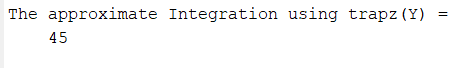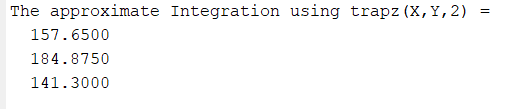Related Articles

# Trapezoidal numerical integration in MATLAB

• Difficulty Level : Medium
• Last Updated : 04 Jul, 2021

Trapz function in MATLAB is used to find the numerical integration using the trapezoidal rule. The basic idea in the Trapezoidal rule is to assume the region under the graph of the given function to be a trapezoid instead of the rectangle and calculate its area.

The formula for numerical integration using trapezoidal rule is:where h = (b-a)/n

MATLAB allows us to perform numerical integration by simply using trapz function instead of going through the lengthy procedure of the above formula.

In MATLAB, trapz function takes the input arguments in 3 different ways.

1. trapz(Y)
2. trapz(X,Y)
3. trapz(_____,dim)

### trapz(Y)

In this method, trapz function considers unit spacing by default. Here Y is numeric data which can be a vector, a matrix, or multidimensional array.

• If Y is a vector, then trapz function computes the approximate integral of Y.
• If Y is a matrix, then trapz function integrates over each column of the matrix and returns a row vector of integration values as output.
• If Y is a multidimensional array, then trapz function integrates over the first dimension whose size does not equal 1.

Let’s take an example for calculating the integral of a vector Y containing function values forin domain [1,5]

Example:

## Matlab

 `% MATLAB code for calculate Y is a vector``% of numeric data containing function values ``% for f(x) = 2^x in domain [1,5]``Y = [2 4 8 16 32];`` ` `% Integrate the data using 'trapz(Y)'``% with unit spacing (by default)``Q = trapz(Y);`` ` `% display the result``disp(``'The approximate Integration using trapz(Y) = '``);``disp(Q);`

Output:### trapz(X,Y)

In this method, X specifies the scalar spacing or the coordinates and trapz function integrates Y with respect to X.

• If X is a vector of coordinates, then the length of X must be equal to the size of the first dimension of Y whose size is not equal to 1.
• If X is scalar spacing, then the value of trapz(X,Y) is equivalent to X*trapz(Y).

Here below example demonstrate the trapz(X,Y) and calculate the integral value of vector Y having a point spacing value of pi/100.

Example:

## Matlab

 `% MATLAB code for calculate the``% integral value of vector Y having``% a point spacing value of pi/100. Where ``% X ranges from 0 to pi/2 with uniform ``% spacing of pi/100``X = 0:pi/100:pi/2;`` ` `% Y is a vector having point spacing ``% value of pi/100``Y = cos(X);`` ` `% Integrate the data using 'trapz(X,Y)'``Q = trapz(X,Y);``disp(``'The approximate Integration using trapz(X,Y) = '``);``disp(Q);`### trapz(______, dim)

Here, dim is the dimension to operate along and in this method, X is an optional argument.

• If dim is specified as 1, then trapz(Y,1) integrates over each column and returns a row vector of integration values.
• If dim is specified as 2, then trapz(Y,2) integrates over each row and returns a column vector of integration values.

The below example Integrates the rows of matrix Y with non-uniform point spacing with dim as 2 by using trapz(_____, dim) function.

Example:

## Matlab

 `% MATLAB code for Integrate the rows of``% matrix Y with non-uniform point spacing``% with dim as 2. Here X contains non-uniform intervals``X = [1 2.5 5.5 10];`` ` `% Y is a matrix  with non-uniform point spacing ``% with value of dim = 2 as data is in the rows of Y ``Y = [13.5   23.7   17.2   13.2;``     ``22.5   16.0  20.5   24.5;``     ``15.6   27.7  10.2   12.9];`` ` `% Integrate the data using 'trapz(X,Y,dim)' ``Q = trapz(X,Y,2);``disp(``'The approximate Integration using trapz(X,Y,2) = '``);``disp(Q);`

Output:My Personal Notes arrow_drop_up